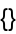#Function Repository Resource:

# ExtractDownValues

Extract all DownValues that match the input

Contributed by: Carl Woll  |  Carl Woll
 ResourceFunction["ExtractDownValues"][expr] finds all DownValues that match expr, in the order in which they would be applied by the pattern matcher. ResourceFunction["ExtractDownValues"][head,args] finds all DownValues of head that match head[args].

## Details and Options

Unless one of the DownValues uses Condition, the first DownValues should be the one that is used by the pattern matcher.

## Examples

### Basic Examples (3)

Define a function:

 In:=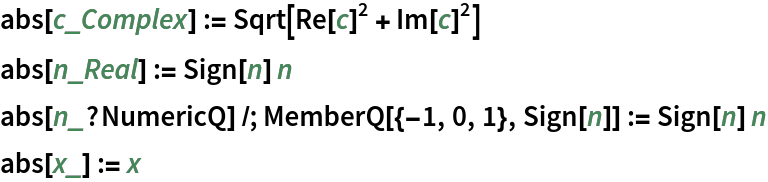Find the DownValues that match abs[23.1]:

 In:=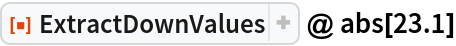Out=Use the two-argument version to find the DownValues that match abs[c] where c=2+I:

 In:=Out=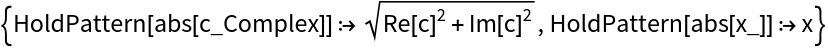### Applications (6)

Find a spoken string:

 In:=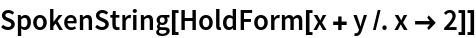Out=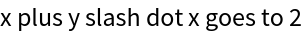Suppose you want to use "where" instead of "slash dot" in this spoken string. Use TracePrint to find the arguments to the internal function SpokenStringDump`SSText used to form the spoken string:

 In:=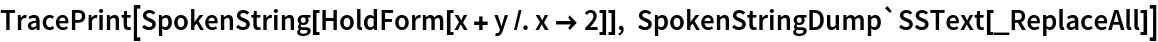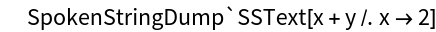Out=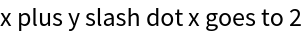The internal function used by SpokenString has many DownValues:

 In:=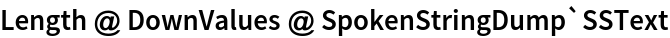Out=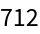ExtractDownValues can find the first downvalue that matches:

 In:=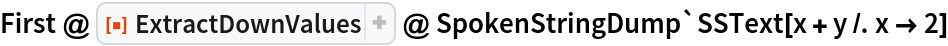Out=Modify this downvalue:

 In:=Check the new output:

 In:=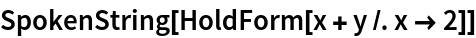Out=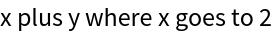### Properties and Relations (2)

When matching patterns, evaluation leaks can occur. Here is a function that has a side effect:

 In:=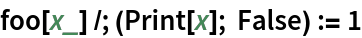Using ExtractDownValues causes the condition to be evaluated:

 In:=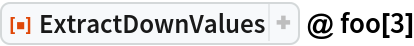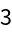Out=